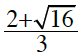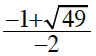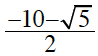### Home > CAAC > Chapter 8 > Lesson 8.2.1 > Problem8-45

8-45.

Calculate the value of each expression below.

1.First find the value of the square root, then solve.

$\frac{2+4}{3}=\frac{6}{3}$

1.First find the value of the square root, then solve.

1.Use the method from part (a).

$\approx -6.1$